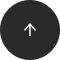Standard SQL

# How to Find Maximum Values in Rows

MAX, WHERE

#### Problem:

You want to find which rows store the largest numeric value in a given column.

#### Example:

Our database has a table named `student` with data in the following columns: `id`, `first_name`, `last_name`, and `grade`.

1LisaJackson3
2GaryLarry5
3TomMichelin2
4MartinBarker2
5EllieBlack5
6MarySimpson4

Let’s find the students who have the highest grades.

#### Solution:

```SELECT  id, first_name, last_name, grade
FROM student
```

Here’s the result:

2GaryLarry5
5EllieBlack5

#### Discussion:

To find the maximum value of a column, use the `MAX()` aggregate function; it takes a column name or an expression to find the maximum value. In our example, the subquery returns the highest number in the column grade (subquery: `SELECT MAX(grade) FROM student`). The main query displays id, first and last name, and their grade. To display only the rows with the maximum value among all values in the column (e.g., `SELECT MAX(grade) FROM student`), use WHERE with a subquery. In WHERE, put the name of the column with the comparable value to the value returned by aggregate function in the subquery(in our example: `WHERE grade = (SELECT MAX(grade) FROM student)`).

#### Recommended articles: